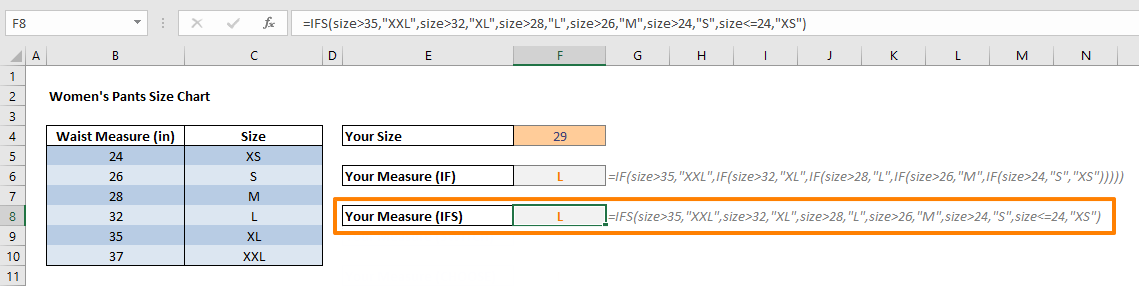Nested IF statements are useful for creating more complicated scenarios in Excel. However, things can get quickly out of hand as the number of conditions increase. For example, five conditions means at least four IF functions, hence lots of parenthesis to deal with. In this guide we're going to go over alternative formulas to use instead of nested IF formulas. Although, they are not seem shorter than multiple IF formulas, you will be only working with a single function. In this guide we're going to see how to simplify nested IF statements.

## IF Function

To perform a logical test, the IF function is usually the go-to method. However, due to its parameter structure, it poses a flaw when it comes to applying more than one logical tests. You need to use nested IF statements in such cases. This can prove challenging to create, read, and maintain. For example you need the following formula to cover possible scenarios in a size chart:

=IF(size>35,"XXL",IF(size>32,"XL",IF(size>28,"L",IF(size>26,"M",IF(size>24,"S","XS")))))Let's now take a look at how you can simplify nested IF formulas by using alternative functions on the same example above.

## IFS and SWITCH

IFS and SWITCH functions have been released in Excel 2016 with Office 365. Each function addresses different pieces of some of the issues stemming from nested IF statements.

The IFS function allows building multiple conditions. On the other hand, SWITCH function accepts only one condition. If you want to return different results based on an expression, use the SWITCH function. If you have different expressions for each level, use the IFS function. Another advantage of the IFS function is that it allows using logic operators like greater than (>) or less than (<) in the expression. SWITCH function only allows exact matching.

Note: You might need an Office 365 subscription to use these functions. Otherwise, you can use the CHOOSE and VLOOKUP functions instead.

### IFS

The IFS function evaluates multiple conditions until it finds the first condition that gives a TRUE result. As the name suggests, it is designed to replace nested IF statements containing multiple IF functions. IFS function’s arguments are test-value pairs.

#### Syntax

=IFS(logical_test1, value_if_true1, [logical_test2, value_if_true2], [logical_test3, value_if_true3],…)

#### Example

=IFS(size>35,"XXL",size>32,"XL",size>28,"L",size>26,"M",size>24,"S",size<=24,"XS")In our example, the IFS function checks for 6 different logical tests in the given order, and returns the corresponding value when it reaches a test result of TRUE. To do this, you would need 5 different IF statements nested inside each other!

### SWITCH

First of all, we recommend that the SWITCH function is not the first option you use for this type scenarios. The function evaluates the value in its first argument against the value-result pairs, and returns the result from the matched pair if there is a match, or the predefined default value if there is none. The SWITCH function is more suitable for scenarios where you need to repeat the expression again and again until you reach the result - not a specific number of conditional statements.

#### Syntax

=SWITCH(expression, value1, result1, [default or value2, result2],…[default or value3, result3])

#### Example

=SWITCH((F4<=40)+(F4<=35)+(F4<=32)+(F4<=28)+(F4<=26)+(F4<=24),1,"XXL",2,"XL",3,"L",4,"M",5,"S",6,"XS")For this scenario, the SWITCH function acts like just a not-so-great imitation of the CHOOSE function. Let's see how this can be done differently with the CHOOSE and VLOOKUP functions.

## CHOOSE and VLOOKUP

The CHOOSE and VLOOKUP functions are a good alternative if you do not have an Office 365 subscription.

### CHOOSE

The CHOOSE function returns a value from the list of arguments based on the specified position. Although, this definition does not sound like what nested-ifs do, you can alter its use to accommodate for this. Let’s see the syntax of the CHOOSE function:

#### Syntax

CHOOSE(index_num, value1, [value2], …)

#### Example

When Excel evaluates a condition, it returns a Boolean value based on the outcome – TRUE if condition is met, and FALSE otherwise. In addition to this, Excel also considers Boolean values to be 1 and 0 if they are in a mathematical operation. For example, the following formula returns 2 because the sum of FALSE, TRUE and TRUE values is equal to 0 + 1 + 1. These met and not met conditions can be used to generate an index number, which points to a value in the arguments of the CHOOSE function.

In the following formula, the same conditions are used for the CHOOSE function's index number. If only 1 condition is met, the formula returns "XXL"; if 2 conditions are met, the formula returns "XL", and so on.

=CHOOSE((F4<=40)+(F4<=35)+(F4<=32)+(F4<=28)+(F4<=26)+(F4<=24),"XXL","XL","L","M","S","XS")### VLOOKUP

Last, but not least is the VLOOKUP function for nested IF statement alternatives. With a tweak on the data set, you can create the shortest formula using the VLOOKUP function. The VLOOKUP function's approximate search mode can be a life saver when you need to work with a range of numbers. Essentially, VLOOKUP searches values for the lookup value. If it finds an exact match, it will use it. If not, VLOOKUP will "step back" and match the previous row. Let's see this in an example.

#### Syntax

VLOOKUP(lookup_value, table_array, col_index_num, TRUE) -> Approximate match

#### Example

For example, in our list, the maximum value of "L" size is 32 inches. This means any number from 29 to 32 corresponds to the "L" size. You can configure the VLOOKUP function to consider any number between 29 and 32 as the lower limit of the range, 29.

To make this arrangement possible, we need to modify the data first. Instead of using maximum values for size letters (28 for "M", 32 for "L"), the list should have the minimum numbers (27 for "M", 29 for "L"). After this adjustment, use the VLOOKUP function in approximate match mode.

=VLOOKUP(size,\$B\$16:\$C\$21,2,TRUE)Please note that VLOOKUP assumes that the first column is sorted either numerically or alphabetically when using approximate match.